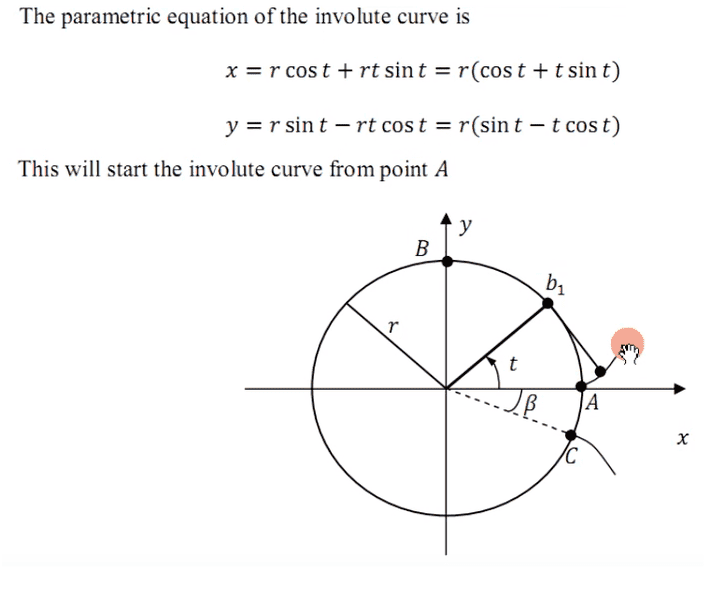# Involute Equation

• I
Does this involute equation is correct?## Answers and Replies

QuantumQuest
Science Advisor
Gold Member
It is the parametric equation of the involute of a circle in Cartesian coordinates.

It is the parametric equation of the involute of a circle in Cartesian coordinates.

Um, I don't know. What do you think it is?

QuantumQuest
Science Advisor
Gold Member
Um, I don't know. What do you think it is?

It is the parametric equation of the involute of a circle in Cartesian coordinates.

•ProfuselyQuarky
rcgldr
Homework Helper
Wiki article:

http://en.wikipedia.org/wiki/Involute#Involute_of_a_circle

This is also the path of an object connected to a string that wraps or unwraps around a circular post. The string would be the black line in the wiki animation. The string is always perpendicular to the instantaneous path of the object, and always tangent to the circular post.

So, does this involute equation is correct?

Why no one can answer my question?

We did answer your question.

Is the " t " in degree or radian?

Radian

OK, now let me work for the equation.

x = r(cos t + t sin t)
y = r(sin t - t cos t)

I start with t = 0 rad and r = 1, then

x = 1(cos 0 + 0 sin 0)
= 1(cos 0)
=1(1)
= 1

y = 1(sin 0 - 0 cos 0)
= 1(sin 0)
= 0

Is it correct?

Yes.